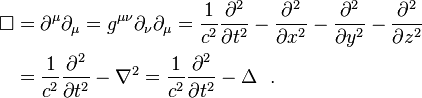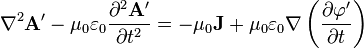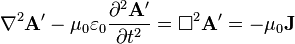# Vector Laplacian

• I
Gold MemberIs on of these pages wrong or I misunderstand it?

I am asking because I want to know what does Δ2 equal to in thisand thisequation on this https://en.wikipedia.org/wiki/Mathematical_descriptions_of_the_electromagnetic_field#Coulomb_gauge page.

Orodruin
Staff Emeritus
Homework Helper
Gold Member
2021 Award
The d'Alembertian is a differential operator. You can apply it to a scalar and get a scalar, or you can apply it to a vector and get a vector.

Gold Member
So since magnetic potential A in this equationis vector ##\nabla^2=(\sum_{i=1}^3(\frac{\partial^2 A}{\partial x_i*\partial x_1});\sum_{i=1}^3(\frac{\partial^2 A}{\partial x_i*\partial x_2});\sum_{i=1}^3(\frac{\partial^2 A}{\partial x_i*\partial x_3}))## not ##\nabla^2=\sum_{i=1}^3(\frac{\partial^2 A}{\partial x_i^2})##?
These are Cartesian coordinates.

Orodruin
Staff Emeritus
Homework Helper
Gold Member
2021 Award
It is unclear what you intend to write with these expressions. In particular, it is unclear what you intend for ##A## to be (the vector, the vector components, etc). I strongly suggest that you do not write vectors on component form (x;y;z), but instead use basis vectors. This will make it much clearer what is intended.

The Laplace operator applied to a vector in Cartesian coordinates is such that the ##x## component of ##\nabla^2 \vec A## is equal to ##\nabla^2 A_x##, where ##A_x## is the ##x## component of ##\vec A##.

•olgerm
Orodruin
Staff Emeritus
Homework Helper
Gold Member
2021 Award
A is vector-field. I am trying to find out what does ##\nabla^2 A##, on this Wikipedia page
https://en.wikipedia.org/wiki/Mathematical_descriptions_of_the_electromagnetic_field#Gauge_freedom , equal to.
Is it that ##(\nabla^2 A)_i=\frac{\partial^2 A_i}{\partial x_i^2}## or ##(\nabla^2 A)_j=\sum_{i=1}^3(\frac{\partial^2 A_i}{\partial x_i \cdot \partial x_j})## or ##\nabla^2 A=\sum_{i=1}^3(\frac{\partial^2 A_i}{\partial x_i^2})##?
Where ##A_i## is the ##i## component of ##\vec A##.
Neither, see my previous post.

Your first option is just one term in the sum, your second is grad(div(A)) and not the Laplacian of A, your third is a scalar.

Gold Member
Yes. Although this is only valid in Cartesian coordinates.
Ok, thanks.